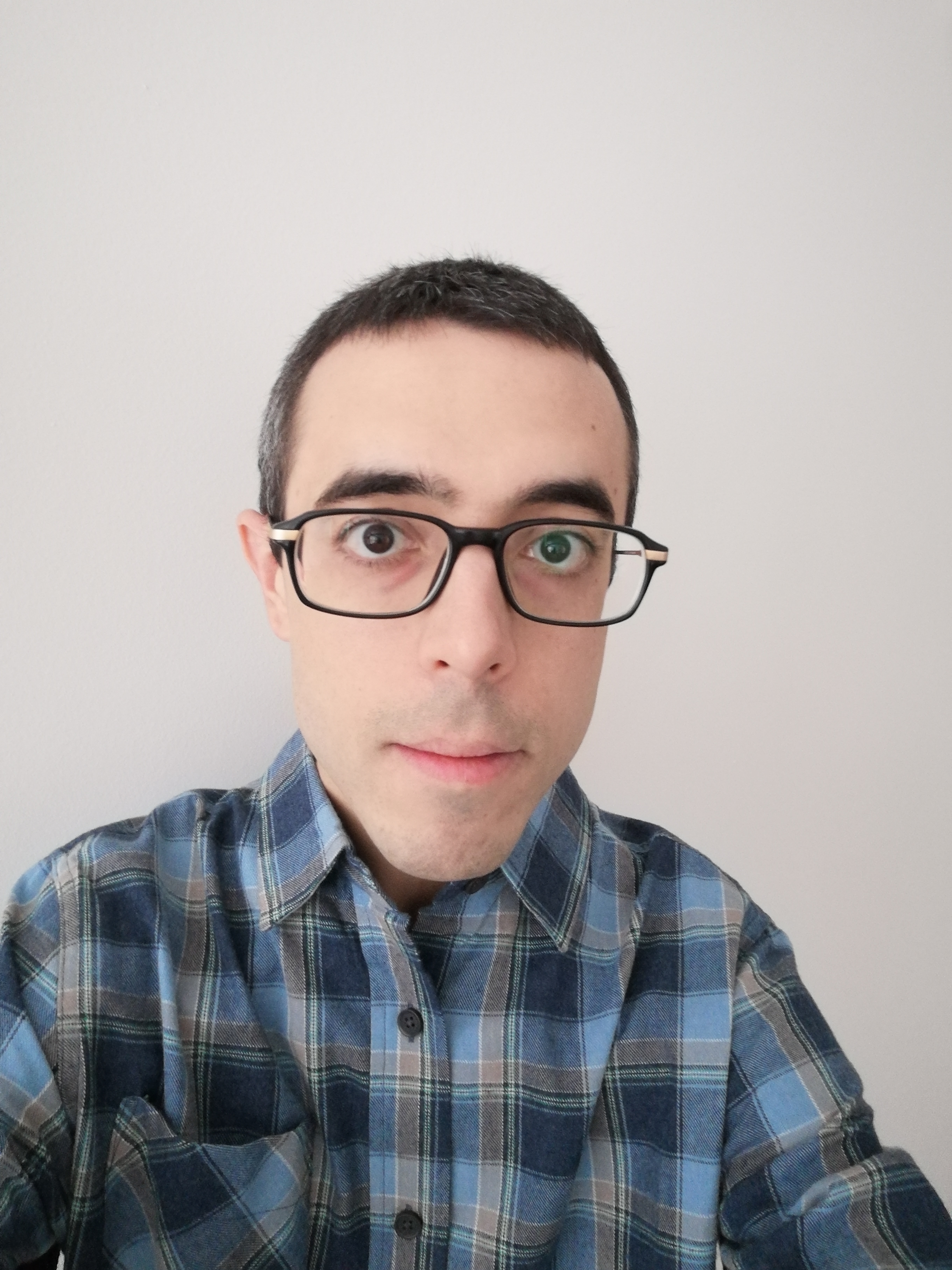# Yiannis LoizidesI am an assistant professor in the Mathematics Department at George Mason University. My research interests are symplectic/Poisson geometry, moduli spaces, loop groups, index theory and noncommutative geometry (in the sense of Alain Connes). Before coming to Mason, I was a postdoc at Cornell (mentored by Reyer Sjamaar) and at Penn State (mentored by Nigel Higson). Before that I was a PhD student in Toronto under the supervision of Eckhard Meinrenken.

GMU students interested in hearing more about possible research topics should reach out. The broad subject areas involved are: differential geometry, functional analysis, Lie theory. Some of what I work on is inspired by physics (although background in physics is not necessary).

E-mail: yloizide `at' gmu `dot' edu

## Papers and preprints

• Norm-square localization for Hamiltonian LG-spaces. J. Geom. and Phys. 114 (2017), 420--449.
• Quantization of Hamiltonian loop group spaces, with Y. Song. Mathematische Annalen 374(1) (2019), 681--722.
• Spinor modules for Hamiltonian loop group spaces, with E. Meinrenken and Y. Song. J. Sympl. Geom. 18(3), (2020), 889--937.
• Quasi-polynomials and the singular [Q,R]=0 theorem. SIGMA 15 (2019), 090, 15 pages.
• Norm-square localization and the quantization of Hamiltonian loop group spaces, with Y. Song. J. Functional Analysis 278(9), (2020).
• Semi-classical analysis of piecewise quasi-polynomial functions and applications to geometric quantization, with P-E. Paradan and M. Vergne, Indag. Math. 32(1), (2021), 151--192.
• Geometric quantization of b-symplectic manifolds, with M. Braverman and Y. Song, J. Sympl. Geom. 19(1), (2021), 1--36.
• Geometric K-homology and the Freed-Hopkins-Teleman theorem, J. Noncommutative Geometry 16(1), (2022), 77--118.
• The decomposition formula for Verlinde sums, with E. Meinrenken, Ann. l'Inst. Fourier 72(3), (2022), 1207--1248.
• A KK-theoretic perspective on deformed Dirac operators, with R. Rodsphon and Y. Song, Advances in Math. 380, (2021).
• Log symplectic manifolds and [Q,R]=0, with Y. Lin, R. Sjamaar, and Y. Song, Int. Math. Res. Not. 2022(18), (2022), 14034--14066.
• Connes-Moscovici residue cocycle for some Dirac-type operators, with A.R.H.S. Sadegh and J. Sanchez, Muenster J. Math. 23, (2023), 25--50.
• Symplectic reduction and a Darboux-Moser-Weinstein theorem for Lie algebroids, with Y. Lin, R. Sjamaar and Y. Song, Pure Appl. Math. Quart. , to appear.
• Differential geometry of weightings, with E. Meinrenken, Advances in Math. 424, (2023).
• Singular Lie filtrations and weightings, with E. Meinrenken, Pure Appl. Math. Quart. , to appear.
• Index formula for Hamiltonian loop group spaces, preprint (2021).
• Families of elliptic boundary problems and index theory of the Atiyah-Bott classes, preprint (2023).
• Verlinde sums and [Q,R]=0, with E. Meinrenken, (2022).
• Riemannian foliations and geometric quantization, with Y. Lin, R. Sjamaar and Y. Song, preprint (2022).
• A fixed-point formula for Dirac operators on Lie groupoids, with A.R.H.S. Sadegh, S. Liu, and J. Sanchez, preprint (2023).

## Slides

CMS summer meeting, June 2019, Quasi-polynomials, asymptotics and [Q,R]=0

Banff workshop on geometric quantization, April 2018, Geometric K-homology and the Freed-Hopkins-Teleman theorem

CMS winter meeting, Dec 2016, [Q,R]=0 and Verlinde series

Penn State, Nov 2016, Quantization of Hamiltonian LG-spaces

Workshop on Geometric Quantization, July 2015, Norm-square localization for Hamiltonian LG-spaces

## Expository notes

Introductory notes on the Atiyah-Hirzebruch spectral sequence pdf

Subelliptic estimates and hypoelliptic Laplacian for T*S^1 pdf

Introduction to gerbes (2015) pdf

Transversally elliptic operators (2013) pdf

Moduli spaces of flat connections with boundary conditions (2012) pdf

Fock-Rosly Poisson structure (2013) pdf

Singularity theorems in GR (2009) pdf